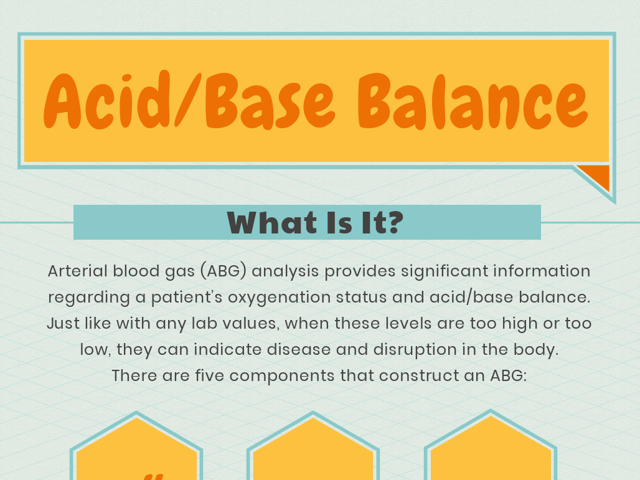# Acid/Base Balance

Arterial blood gas (ABG) analysis provides significant information regarding a patient’s oxygenation status and acid/base balance. Just like with any lab values, when these levels are too high or too low, they can indicate disease and disruption in the body. There are five components that construct an ABG: blood acidity (pH), partial pressure of oxygen ($$PaO_2$$), partial pressure of carbon dioxide ($$PaCO_2$$), bicarbonate ($$HCO_3$$), and oxygen saturation ($$O_2$$). The normal range for each component is listed below:

pH: 7.35–7.45
$$PaO_2$$: 75–100 mmHg
$$PaCO_2$$: 35–45 mmHg
$$HCO_3$$: 22–26 mEq/L
$$O_2$$: 94–100%

## What Do the Lab Values Tell Us?

When the blood pH is less than 7.35, the blood is acidotic. If the blood pH is higher than 7.45, the blood is considered alkalotic. Using the pH results with the $$CO_2$$ and $$HCO_3$$ markers, it can be determined if the patient’s acidosis or alkalosis is respiratory or metabolic in nature. The $$CO_2$$ value directly relates to the respiratory system, while $$HCO_3$$ relates to the metabolic system. An elevated $$CO_2$$ with a decreased pH indicates respiratory acidosis, while a decreased $$CO_2$$ with an increased pH indicates respiratory alkalosis. A decreased $$CO_2$$ with a decreased pH indicates metabolic acidosis, while an increased $$CO_2$$ with an increased pH indicates metabolic alkalosis.

## How Can I Remember This?

An easy way to remember which imbalances go with which diagnosis is the mnemonic ROME. ROME stands for Respiratory Opposite, Metabolic Equal. It focuses on the relationship between the pH and carbon dioxide and bicarbonate markers. In respiratory conditions, the pH and $$CO_2$$ are inversely related, whereas in metabolic conditions the pH and $$CO_2$$ are directly related. This can be seen below:

R - Respiratory
O - Opposite

• pH < 7.35, $$PaCO_2$$ > 45 mmHg = respiratory acidosis
• pH > 7.45, $$PaCO_2$$ < 35 mmHg = respiratory alkalosis

M - Metabolic
E - Equal

• pH < 7.35, $$HCO_3$$ < 22 mEq = metabolic acidosis
• pH > 7.45, $$HCO_3$$ > 26 mEq = metabolic alkalosis

Understanding these values helps in interpreting the patient’s condition and the best mechanisms of treatment. The information provided by the ABG further supports many diagnoses related to specific disorders associated with blood acid/base imbalance.